# Section 06: pvalues

Notes & Jupyter Notebook Aoyue Mao (2021), adapted from Danylo Lavrentovich, Irina Shlosman, Kevin Mizes notes from past years

# Week 06 section

In this week's lecture, we learned what p-values exactly mean. They tell us given some null hypothesis on how data is generated, how surprising a particular observation is.

This section will not focus on p-values. Instead, it will cover general features of parameter estimation, review Bayes rule, introduce the T distribution, and provide small hint for the p-set.

There is also a Jupyter notebook that covers all of the above more densely, along with practice problems, an example p value calculation, and some interactive functions that you can run/modify to explore null vs. alternative hypotheses, visualize estimation procedures, etc. Here's the notebook: w06_section.ipynb. To run the pre-written functions you need to download this helper file: w06_section_utils.py.

## Parameter Estimation/Inference

Given some data $$D=\{X_1,X_2,\ldots,X_i\}$$, we'd like to describe the process that produced the data. Here are two example scenarios with different probability distributions.

### Example 1: Weights of Siberian huskies (Normal)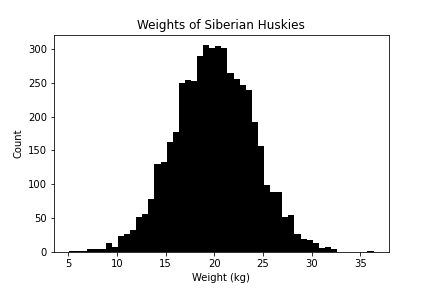Weight is a continuous random variable. Based on our experience, it follows a normal distribution. Thus, the parameter estimation question is to ask: what particular $$\mu, \sigma$$ describe the data $$D$$?

### Example 2: Number of heads out of $$N$$ flips of a coin (Binomial)

Every time we flip a coin, we get a heads with probability $$p$$, or a tails with probability $$1-p$$. We flipped the coin $$N=30$$ times. #heads is a discrete random variable and it follows the binomial distribution.

We got 25 heads. We didn't know whether the coin was fair or not. So we can ask: what particular $$p$$ describes the number of heads?

### Notation

We use $$\theta$$ to denote a hypothesis on how the data is generated. It is a set of particular values of the parameters of the underlying probability distribution. It may contain one or more parameters.

For dog weights (normal): $$\rm \theta=\{\mu=20\ kg,\sigma=4\ kg\}$$;

For an unbiased coin (binomial): $$\theta=\{p=0.5\}$$.

The big question we'd like to answer:

Given data $$D$$, what is the most probable $$\theta$$?

### Reviewing Bayes rule

To pick out a most probable $$\theta$$, let's first define a set of hypotheses. Suppose we have a set of $$M$$ hypotheses, $$\theta_1, \dots, \theta_M$$.

Given data, how probable is a particular hypothesis $$\theta_k$$?

This sounds like a conditional probability... which means it's time to stare at Bayes rule:

$$P(\theta_k \mid D) = \frac{P(D\mid \theta_k)P(\theta_k)}{P(D)}$$

Let's review the terms:

• $$P(\theta_k \mid D)$$ is the posterior. How probable is the hypothesis post seeing the data?
• $$P(D\mid \theta_k)$$ is the likelihood. How probable is the data, given that the hypothesis is true?
• $$P(\theta_k)$$ is the prior. How probable is the hypothesis, prior to seeing the data?
• $$P(D)$$ is the marginal. Or it might be called a normalization factor. How probable is the data under all possible hypotheses?

### Reviewing marginalization

\begin{aligned} P(D) &= P(D \text{ and }\theta_1) + P(D \text{ and }\theta_2) + \dots + P(D \text{ and }\theta_M) \\ &= \sum_{i=1}^M P(D \text{ and }\theta_i) \\ &= \sum_{i=1}^M P(D \mid \theta_i) P(\theta_i) \\ \end{aligned}

Here we've assumed there's a finite number of hypotheses. If we have an infinite number, you could see the marginal written as something like $$P(D) = \int P(D \mid \theta') P(\theta') d\theta'$$.

### Practice Problem: Mosquito's Blood Preference

ABO blood types exist in different frequencies in the human population. (At least some say that) they also attract mosquitos differently.

Blood type Frequency in population Mosquito landing chance
A 0.42 0.453
B 0.10 0.569
AB 0.04 0.480
O 0.44 0.785

Shirai et al (2004)

Compute the following terms.

• $$P(\text{O type})$$
• $$P(\text{AB type})$$
• $$P(\text{Landing}\mid \text{B type})$$
• $$P(\text{Not landing}\mid \text{A type})$$
• $$P(\text{Landing})$$
• $$P(\text{O type}\mid\text{Landing})$$
• $$P(\text{AB type}\mid\text{Not landing})$$

### Comparing two hypotheses

I forgot my exact blood type, but I'm sure it is either A or O. And I was bitten by a mosquito today. How is this reflected in the posteriors?

$$P(\text{A type}\mid \text{Landing})=\frac{P(\text{Landing}\mid \text{A type})P(\text{A type})}{P(\text{Landing})}$$
$$P(\text{O type}\mid \text{Landing})=\frac{P(\text{Landing}\mid \text{O type})P(\text{O type})}{P(\text{Landing})}$$

To compare these two hypotheses, I can take a ratio of their posterior probabilities. The denominator $$P(\text{Landing})$$ cancels out.

Generally speaking, when we look for the hypothesis with the highest $$P(\theta \mid D)$$, we can ignore the denominator, since it's unaffected by hypothesis.

• If we have some prior beliefs $$P(\theta)$$, the $$\theta$$ that maximizes $$P(D\mid \theta)P(\theta)$$ is the maximum a posteriori (MAP) estimation.
• If we further assume uniform priors $$P(\theta)$$ on all $$\theta$$, then $$P(\theta \mid D) \propto P(D\mid \theta)$$. Now, the $$\theta$$ that maximizes $$P(D\mid \theta)$$ (a.k.a. likelihood) is the maximum likelihood estimation (MLE).

## Concepts behind likelihood $$P(D \mid \theta)$$ with binomial

Binomial process: we run $$n$$ trials, with each trial having a success probability $$p$$, and we count how many successes we get out of the $$n$$ trials.

The probability mass function: (The probability of getting $$k$$ successes)

$$P(k \mid p, n) = {n \choose k} p^k (1-p)^{n-k}$$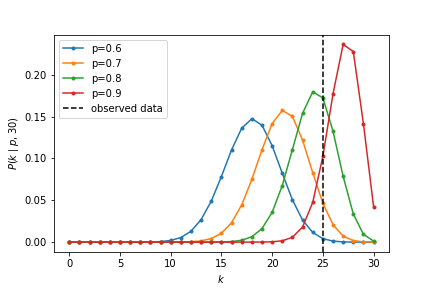The likelihood $$P(k=25 \mid n=30,p)$$ depends on $$p$$. We can do MLE analytically in this binomial case. A convenient trick that comes up frequently with this kind of problem is to maximize the log likelihood instead of the likelihood. This is okay to do because the logarithm is a monotonically increasing function (the $$x$$ that maximizes $$f(x)$$ also maximizes $$\log f(x)$$). Exponents come up a lot in statistics formulas, and taking the log helps to separate them out.

The log likelihood:

\begin{aligned} \log L(p) &= \log \bigg[ {n \choose k}p^k (1-p)^{n-k}\bigg] \\ &= \log {n \choose k}+ k \log(p) + (n-k)\log(1-p) \end{aligned}

Now it's a little easier to take the derivative with respect to $$p$$:

\begin{aligned} \frac{\partial}{\partial p} \log L(p) &= \frac{\partial}{\partial p} \bigg[ \log {n \choose k}+ k \log(p) + (n-k)\log(1-p) \bigg] \\ &= \frac{k}{p} - \frac{n-k}{1-p} \end{aligned}

Setting it to zero to find our maximizer, $$\hat{p}$$ (you should check it's a maximum by taking the second derivative too):

$$\frac{k}{p} - \frac{n-k}{1-p} = 0 \implies k - kp = np - kp \implies \hat{p} = \frac{k}{n}$$

Rather unsurprisingly, if we observe $$k$$ heads out of $$n$$ flips, the maximum likelihood estimate of the probability of heads is just $$k/n$$.

We can also plot $$\log L(p)$$ against $$p$$. Clearly, there exists a maximum.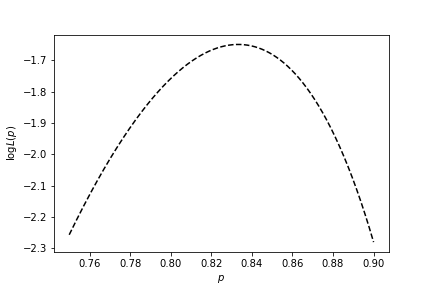We find the probability mass function curve for this MLE. It indeed has the most overlap with the observed data.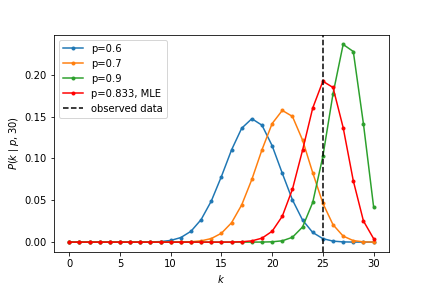A few things to consider: - Does this mean the null hypothesis (fair coin) is invalidated? - Does this mean $$p=k/n$$ has to be the correct model? - What if we had (non-uniform) prior beliefs, $$P(\theta)$$? Maybe we really tend to trust the coin is fair...?

## MLE for the normal distribution

Now let's switch gears to something a little more involved. Suppose we observe $$n$$ data points $$x_1, \dots, x_n \sim N(\mu, \sigma)$$. Just as we did for binomial distribution, let's try to maximize the likelihood of the observed data with respect to the parameters that describe it.

For a single point $$x$$, the normal probability density function (pdf for continuous distributions; pmf for discrete distributions) is

$$P(x| \mu, \sigma^2) = \frac{1}{\sqrt{2\pi\sigma^2}} e^{-\frac{(x-\mu)^2}{2\sigma^2}}$$

The joint probability of $$n$$ points, assuming they are all independently, identically distributed:

\begin{aligned} P(x_1, \dots, x_n \mid \mu, \sigma^2) &= P(x_1 \mid \mu, \sigma^2)\cdot P(x_2 \mid \mu, \sigma^2)\dots P(x_n \mid \mu, \sigma^2) && \text{assume data is IID} \\ &= \prod_{i=1}^n P(x_i \mid \mu, \sigma^2) && \text{use index notation} \\ &= \prod_{i=1}^n \frac{1}{\sqrt{2\pi\sigma^2}} \exp\big(-\frac{(x_i-\mu)^2}{2\sigma^2} \big) && \text{plug in PDF}\\ &= \bigg(\frac{1}{\sqrt{2\pi\sigma^2}}\bigg)^n \times \bigg[ \prod_{i=1}^n \exp\big(-\frac{(x_i-\mu)^2}{2\sigma^2} \big) \bigg] && \text{pop out the constant from the product}\\ &= \big(2\pi\sigma^2\big)^{-\frac{n}{2}} \times \bigg[ \prod_{i=1}^n \exp\big(-\frac{(x_i-\mu)^2}{2\sigma^2} \big) \bigg]&& \text{rewrite constant a little bit} \\ &= \big(2\pi\sigma^2\big)^{-\frac{n}{2}} \times \exp\bigg( -\sum_{i=1}^n \frac{(x_i-\mu)^2}{2\sigma^2} \bigg) && \text{product of exps is exp of sum}\\ \end{aligned}

At this point it will be convenient to take the log again:

\begin{aligned} \log P(x_1, \dots, x_n \mid \mu, \sigma^2) &= \log \bigg[ \big(2\pi\sigma^2\big)^{-\frac{n}{2}} \times \exp\bigg( -\sum_{i=1}^n \frac{(x_i-\mu)^2}{2\sigma^2} \bigg) \bigg] \\ &= -\frac{n}{2}\log\big(2\pi\sigma^2\big) + \log \bigg[ \exp \bigg( -\sum_{i=1}^n \frac{(x_i-\mu)^2}{2\sigma^2} \bigg) \bigg] \\ &= -\frac{n}{2}\log\big(2\pi\sigma^2\big) - \sum_{i=1}^n \frac{(x_i-\mu)^2}{2\sigma^2} \\ \end{aligned}

#### MLE of $$\mu$$

\begin{aligned} \frac{d}{d\mu} \log P(x_1, \dots, x_n\mid \mu, \sigma^2) &= 0 \\ \frac{d}{d\mu} \bigg( -\frac{n}{2}\log\big(2\pi\sigma^2\big) - \sum_{i=1}^n \frac{(x_i-\mu)^2}{2\sigma^2} \bigg ) &= 0 \\ \sum_{i=1}^N \frac{-\frac{d}{d\mu} (x_i-\mu)^2}{2\sigma^2} &= 0 \\ \sum_{i=1}^N \bigg( \frac{x_i-\mu}{\sigma^2} \bigg) &= 0 \end{aligned}

Multiplying both sides by $$\sigma$$ and rearranging gives us our likelihood-maximizing $$\mu$$:

$$\hat{\mu} = \frac{\sum_{i=1}^n x_i}{n}$$

The maximum likelihood estimate of the mean given some observed $$x_i$$'s is the sample mean.

#### MLE of $$\sigma^2$$

\begin{aligned} \log P(x_1, \dots, x_n \mid \mu, \sigma^2) = \log L(\mu, \sigma^2) = -\frac{n}{2}\log\big(2\pi\sigma^2\big) - \sum_{i=1}^n \frac{(x_i-\mu)^2}{2\sigma^2} \\ \end{aligned}

Taking derivative wrt $$\sigma^2$$:

$$\frac{\partial}{\partial\sigma^2} \log L(\mu, \sigma^2) = -\frac{n}{2\sigma^2} + \sum_{i=1}^n \frac{(x_i-\mu)^2}{2(\sigma^2)^2} = 0$$

Skipping some steps here (it is a good exercise to do these calculations by hand), we arrive at our estimate:

$$\hat{\sigma^2} = \frac{1}{n}\sum_{i=1}^n (x_i-\mu)^2$$

This expression is not too surprising -- it's the average squared distance from the mean over all data points.

### Estimation of Gaussian parameters only given data... the road to the T-distribution

Let's say we observe some small number of data points $$x_1, \dots, x_n$$ (around $$n=5$$) that we assume are generated by a Gaussian process, $$x_1, \dots, x_n \sim N(\mu, \sigma^2)$$, and we'd like to use the data to estimate $$\mu$$ and $$\sigma$$.

From our analysis above, - the maximum likelihood estimator for the mean is the sample mean: $$\hat{\mu} = \bar{x} = \frac{1}{N} \sum_{i=1}^n x_i$$ - the maximum likelihood estimator for the variance is: $$\hat{\sigma^2} = \frac{1}{n} \sum_{i=1}^n (x_i - \mu)^2$$

Wait a second. The MLE for the variance includes $$\mu$$, something we're trying to estimate with $$\bar{x}$$!

If we plug in $$\bar{x}$$ for $$\mu$$ to get a new estimator for the variance, $$\hat{\sigma^2} = \frac{1}{n} \sum_{i=1}^n (x_i - \bar{x})^2$$, it turns out that this estimator is biased. An estimator $$\hat{\theta}$$ of a parameter $$\theta$$ is a function of data and is biased if the expected value of $$\hat{\theta}$$ over all possible data is not exactly $$\theta$$. A small correction is needed to yield an unbiased estimator of the population variance: $$\frac{1}{n-1}$$ rather than $$\frac{1}{n}$$. This is the Bessel correction -- you can read more about it here and here.

So, given some data, an unbiased estimator of the population variance is the (Bessel-corrected) sample variance, $$S^2 = \frac{1}{n-1}\sum_{i=1}^n (x_i - \bar{x})^2$$

## Hypothesis testing on $$\bar{x}$$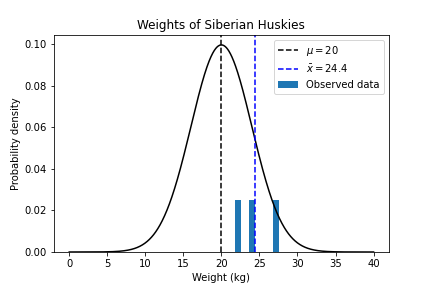Q: How likely is it that we'd get this particular $$\bar{x}$$ value?

$$\bar{x}$$ has a variance of $$\frac{\sigma^2}{n}$$ (refer to the detailed Jupyter notebook for proof); so the "standard error of the mean" is $$\frac{\sigma}{\sqrt{n}}$$. $$\bar{x}$$ is then turned into a standard score:

$$\frac{\bar{x}-\mu}{\sigma/\sqrt{n}}$$
• If $$\sigma$$ is known, this is a Z score
$$Z=\frac{\bar{x}-\mu}{\sigma/\sqrt{n}}$$

The Z score also follows a normal distribution.

• If $$\sigma$$ is unknown, we estimate it with $$s$$ (sample standard deviation). This becomes a T score
$$T=\frac{\bar{x}-\mu}{s/\sqrt{n}}$$

with $$n-1$$ degrees of freedom, and it no longer follows the nornal distribution. Instead, it follows a Student's t distribution with $$n-1$$ degrees of freedom.

### Distributions of Z and T scores

https://www.scribbr.com/statistics/t-distribution/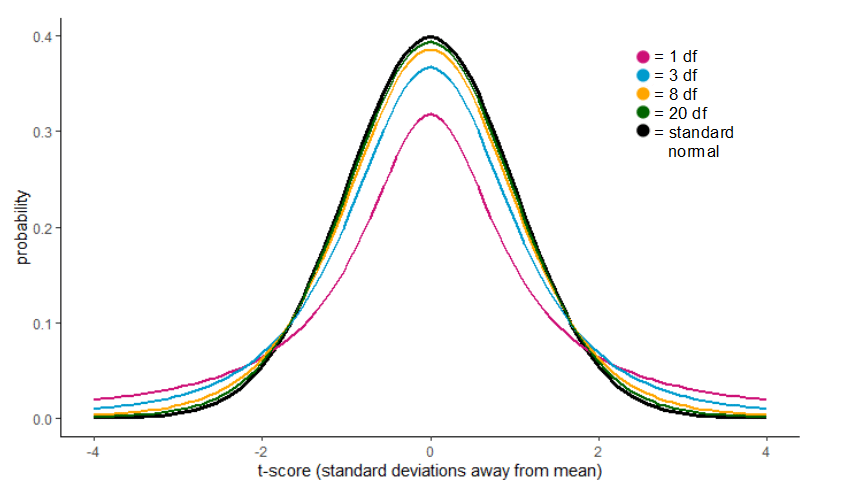• T distribution has fatter tails. It allows for $$\bar{x}$$ to be "far away" from $$\mu$$ because we had to estimate $$\sigma^2$$ from the data.
• We could have estimated too small a $$\sigma^2$$, which means the T score would be larger than it should be. Hence, more probability of big T scores.
• At large $$n$$, we estimate $$\sigma^2$$ well, so T distribution converges to Z distribution.

So T distributions can arise from estimation of an unknown $$\sigma^2$$ given data. In the pset, we will marginalize over many potential $$\sigma^2$$.

## Concepts for pset

Inferring $$(\mu,\sigma)$$ of a normal distribution. The true values are from finite choices. For each candidate $$(\mu,\sigma)$$, calculate the posterior $$P(\mu,\sigma \mid x_1, \ldots, x_n)$$.